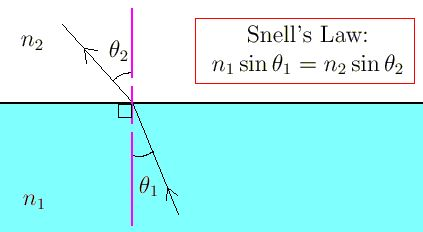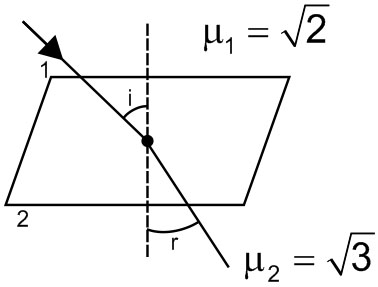# Let the plane be the boundary between two transparent media. Medium 1 in has a refractive index of and medium 2 with has a refractive index of . . A ray of light in medium 1 given by the vector is incident on the plane of separation. The angle of refraction in medium 2 is Option 1) Option 2) Option 3) Option 4)

P Prateek Shrivastava

As we learnt in

Relation between angle of incidence and angle of refaction -

- whereinrefractive index of medium of incidence.

refractive index of medium where rays is refracted.

angle of incidence.

angle of refraction.From Snell's law

Correct option is 2.

Option 1)

This is an incorrect option.

Option 2)

This is the correct option.

Option 3)

This is an incorrect option.

Option 4)

This is an incorrect option.

Exams
Articles
Questions